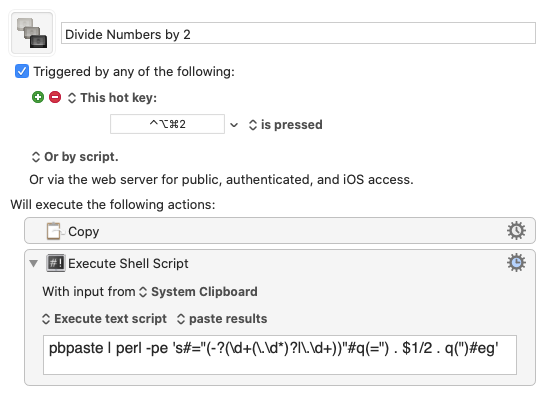# Find every number in a long document and divide it by 2

Hi!

I have a very long text document (a plist for an audio plug-in) that I want to divide every number in it in half.

With the kind help of this forum, I have a macro now where I can highlight a number, trigger the macro, and it will divide in half.

Now I would like to add the ability to put my cursor at the very start of this long text document, trigger the macro, and it would find every number in it and divide the number in half.

I've tried for allot of hours to make this work but is unfortunately way beyond my skills.

The numbers all seem to be in quotes in this particular text document.

Here is a short excerpt from the text document to show what it looks like;

Here is my current macro;

and here is the actual Keyboard Maestro macro file;

Divide by 2.kmmacros (1.9 KB)

Many thanks!!!

Try this:The shell script is a pipeline with an admittedly complicated but really cool Perl one-liner:

``````pbpaste | perl -pe 's#="(-?(\d+(\.\d*)?|\.\d+))"#q(=") . \$1/2 . q(")#eg'
``````

The macro assumes the following:

1. You’ve selected all the text you want to process.
2. All of the numbers you want to divide by two are in a `=”<number>“` format.

The first step copies the selected text to the system clipboard. The second sends the clipboard to the complicated Perl command and then pastes Perl’s output, which replaces the selected text.

The Perl script goes through the text line by line and replaces each instance of

``````="<number>"
``````

with

``````="<number/2>"
``````

It does not change any other numbers in the text, as you would not want something like

``````image_no_peaks="backgrounds/itu/background3.png"
``````

to turn into

``````image_no_peaks="backgrounds/itu/background1.5.png"
``````

The command that does all the work takes advantage of a few of Perl’s powerful but terse features.

• At it’s most basic, it’s a substitute command, which usually looks like

``````s/find/replace/
``````

But if you need to use the slash command for something else, Perl allows you to use other characters, like the hash:

``````s#find#replace#
``````
• What we’re trying to find is a number, which can look like 23, -23, 23.5, .5, -.5, and so on. The regular expression

``````(-?(\d+(\.\d*)?|\.\d+))
``````

does that. There might be better ways to describe a (possibly decimal) number, but that one works for the examples I’ve tested it on.

• By including the `e` flag at the end of the regular expression, Perl knows to evaluate the replacement as if it were a Perl expression. Therefore, the

``````\$1/2
``````

part of the replacement divides the found number by 2. Unfortunately, what would be a very simple replacement gets dirtied up by the need to include the leading `="` and the trailing `"` in the replacement. That’s why the replacement pattern becomes the ugly

``````q(=") . \$1/2 . q(")
``````

the `q` function acts like single quotation marks and, like the hash marks we used earlier, is useful when the thing we’re quoting already has quotation marks in it (or when the expression is already surrounded by single quotation marks). The `.` is Perl’s way of concatenating strings.

• By including the `g` flag at the end of the regular expression, Perl knows to make the substitution for every instance on every line.

Phew! As you might expect, this took a lot longer to explain than it did to write.

I tested it on

``````<settings>
<thing_segment height="-5" />
<thing_color_red hue="0.00" saturation="1.00" brightness="0.97" />
<thing_color_amber7 hue="0.18" saturation="1" brightness="-0.97" />
<thing_color_green hue=".30" saturation="1.0" brightness=".97" />
<v_color_non hue="0.30" saturation="1.00" brightness="0.97" image_no_peaks="backgrounds/itu/background3.png />
<meter_over x="13" y="74" />
<thing_thing x="52" y="79" segment_width="24" vertical="true" />
</settings>
``````

which was changed to

``````<settings>
<thing_segment height="-2.5" />
<thing_color_red hue="0" saturation="0.5" brightness="0.485" />
<thing_color_amber7 hue="0.09" saturation="0.5" brightness="-0.485" />
<thing_color_green hue="0.15" saturation="0.5" brightness="0.485" />
<v_color_non hue="0.15" saturation="0.5" brightness="0.485" image_no_peaks="backgrounds/itu/background3.png />
<meter_over x="6.5" y="37" />
<thing_thing x="26" y="39.5" segment_width="12" vertical="true" />
</settings>
``````
2 Likes

Here's another way to accomplish the same thing with native KM actions (like @drdrang's excellent solution, this macro also assumes you've selected all the text you want the macro to act on before running it, and that the numbers are surrounded by quotes):

This one takes advantage of KM's ability to use regex capture groups in calculation functions, which lets us search the document for numbers in quotes and replace them with that same number divided by half (essentially the same thing @drdrang's macro does, just in a different way).

5 Likes

This is definitely better because it's more native to KM and requires less explanation. I would never have thought of this because I didn't know about the CALCULATE function until now.

Minor note: My regular expression for matching numbers is more complicated because I wanted to avoid matching things like `version="2.1.3"`, which yours will match and try to divide. It's likely never to show up, but I tend to go overboard because I've been burned by things I thought were impossible.

2 Likes

Good point.

Great solution, Gabe.
I really prefer native KM Regex solutions, because they are much easier to dev, test, and share.

This Regex should fix the issue @drdrang raises:
`"(\-?\d*\.?\d*)"`

3 Likes

THANKS SO MUCH !!!!!!!!!!!!!!!!!!!

I try to fake my way through getting these things to work, and just could not get this one.

Very kind of you to take the time to help me!

1 Like